Email us to get an instant 20% discount on highly effective K-12 Math & English kwizNET Programs!

#### Online Quiz (WorksheetABCD)

Questions Per Quiz = 2 4 6 8 10

### High School Mathematics11.14 Principal Diagonal of a Square Matrix, Scalar Matrix and Identity Matrix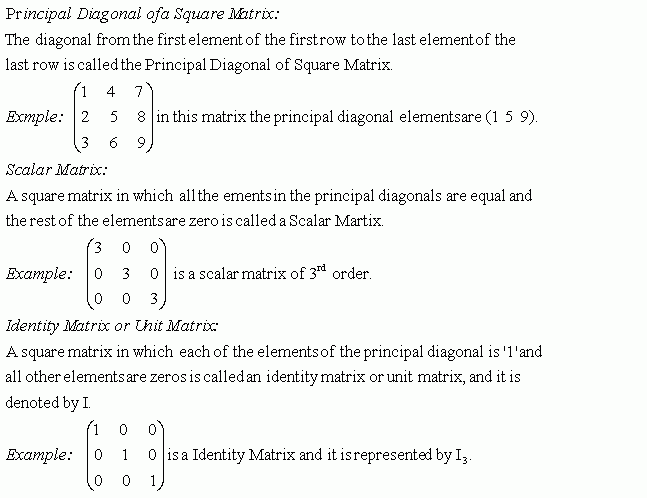Directions: Choose the correct answer. Also write at least 10 examples of your own.
 Q 1: This is a unit matrix of 3rd order, correct it.CorrectNot correct Q 2: Write the principal diagonal elements in the following matrix.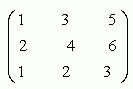(1 2 1)(3 4 2)(5 6 3)(1 4 3) Q 3: This is a scalar matrix, correct it.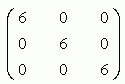CorrectNot correct Q 4: This is a scalar matrix, correct it.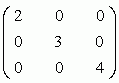Not correctCorrect Q 5: This is a scalar matrix, correct it.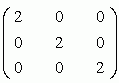CorrectNot correct Q 6: Write the principal diagonal elements in the following matrix.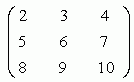(3 6 9)(2 5 8)(2 6 10)(4 7 10) Question 7: This question is available to subscribers only! Question 8: This question is available to subscribers only!

#### Subscription to kwizNET Learning System offers the following benefits:

• Unrestricted access to grade appropriate lessons, quizzes, & printable worksheets
• Instant scoring of online quizzes
• Progress tracking and award certificates to keep your student motivated
• Unlimited practice with auto-generated 'WIZ MATH' quizzes